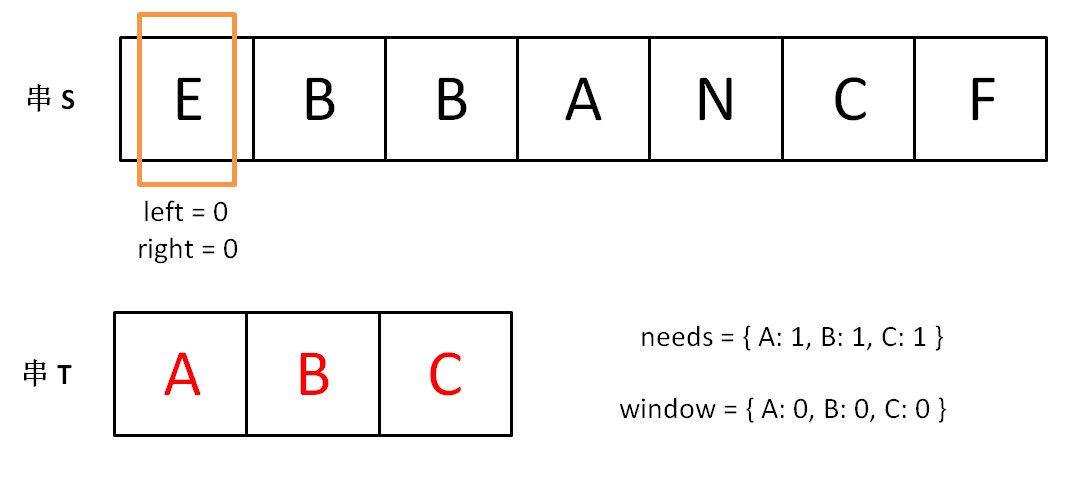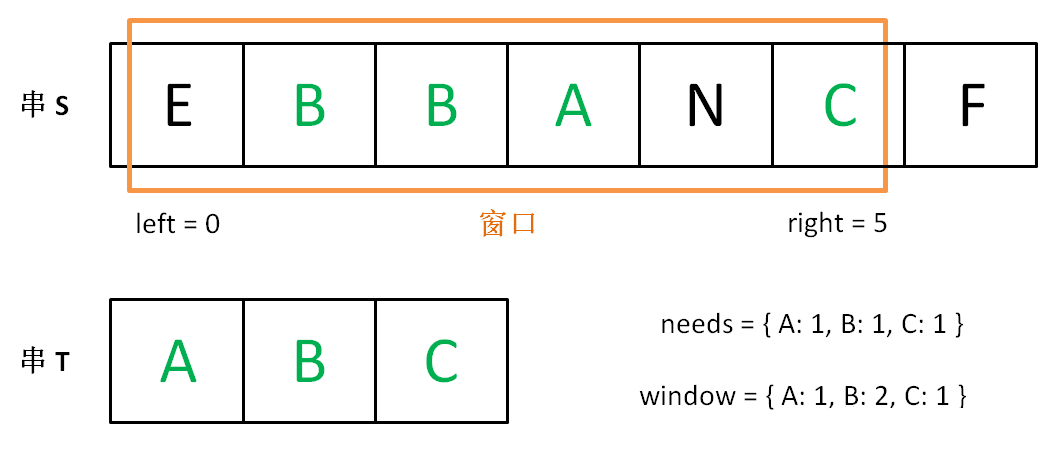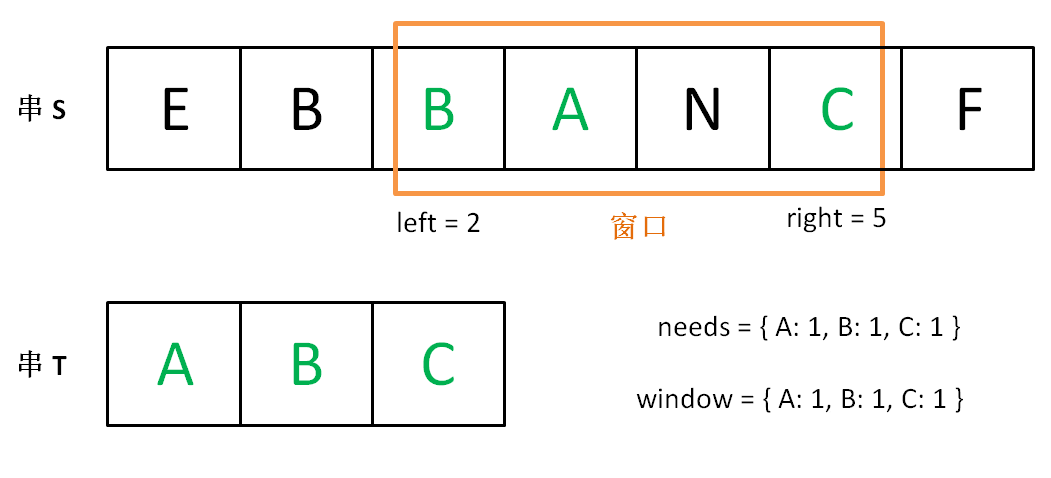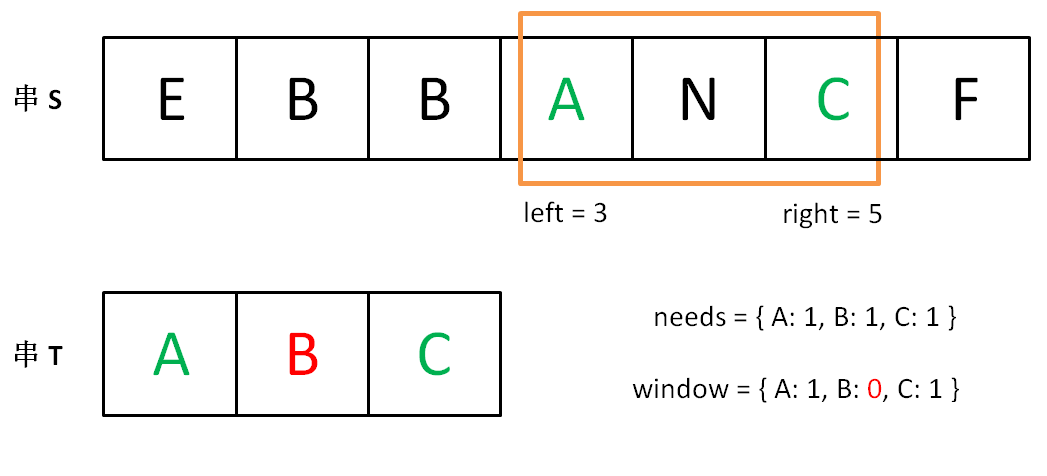# leetcode 76——最小覆盖子串

输入: S = "ADOBECODEBANC", T = "ABC"



• 如果 S 中不存这样的子串，则返回空字符串 “”。
• 如果 S 中存在这样的子串，我们保证它是唯一的答案。

for (int i = 0; i < s.size(); i++)
for (int j = i + 1; j < s.size(); j++)
if s[i:j] 包含 t 的所有字母:
更新答案


1. 我们在字符串 S 中使用双指针中的左右指针技巧，初始化 left = right = 0，把索引闭区间 [left, right] 称为一个「窗口」。
2. 我们先不断地增加 right 指针扩大窗口 [left, right]，直到窗口中的字符串符合要求（包含了 T 中的所有字符）。
3. 此时，我们停止增加 right，转而不断增加 left 指针缩小窗口 [left, right]，直到窗口中的字符串不再符合要求（不包含 T 中的所有字符了）。同时，每次增加 left，我们都要更新一轮结果。
4. 重复第 2 和第 3 步，直到 right 到达字符串 S 的尽头。

needs 和 window 相当于计数器，分别记录 T 中字符出现次数和窗口中的相应字符的出现次数。string s, t;
// 在 s 中寻找 t 的「最小覆盖子串」
int left = 0, right = 0;
string res = s;

while(right < s.size()) {
right++;
// 如果符合要求，移动 left 缩小窗口
while (window 符合要求) {
// 如果这个窗口的子串更短，则更新 res
res = minLen(res, window);
window.remove(s[left]);
left++;
}
}
return res;


string s, t;
// 在 s 中寻找 t 的「最小覆盖子串」
int left = 0, right = 0;
string res = s;

// 相当于两个计数器
unordered_map<char, int> window;
unordered_map<char, int> needs;
for (char c : t) needs[c]++;

// 记录 window 中已经有多少字符符合要求了
int match = 0;

while (right < s.size()) {
char c1 = s[right];
if (needs.count(c1)) {
window[c1]++; // 加入 window
if (window[c1] == needs[c1])
// 字符 c1 的出现次数符合要求了
match++;
}
right++;

// window 中的字符串已符合 needs 的要求了
while (match == needs.size()) {
// 更新结果 res
res = minLen(res, window);
char c2 = s[left];
if (needs.count(c2)) {
window[c2]--; // 移出 window
if (window[c2] < needs[c2])
// 字符 c2 出现次数不再符合要求
match--;
}
left++;
}
}
return res;


string minWindow(string s, string t) {
// 记录最短子串的开始位置和长度
int start = 0, minLen = INT_MAX;
int left = 0, right = 0;

unordered_map<char, int> window;
unordered_map<char, int> needs;
for (char c : t) needs[c]++;

int match = 0;

while (right < s.size()) {
char c1 = s[right];
if (needs.count(c1)) {
window[c1]++;
if (window[c1] == needs[c1])
match++;
}
right++;

while (match == needs.size()) {
if (right - left < minLen) {
// 更新最小子串的位置和长度
start = left;
minLen = right - left;
}
char c2 = s[left];
if (needs.count(c2)) {
window[c2]--;
if (window[c2] < needs[c2])
match--;
}
left++;
}
}
return minLen == INT_MAX ?
"" : s.substr(start, minLen);
}


10-11421604-202295
08-054788
03-0639
07-16240
03-1191
06-161231
08-28345
07-1625
03-0277